The radioactivity of a substance is measured by how many decays per unit time occur. Two popular units of the activity are used: the curie (Ci), defined as

 1 Ci = 3.7 x 1010 decays/s (8)

and the becquerel (Bq), defined as

 1 Bq = 1 decay/s (9)

Note that, by themselves, these units do not measure accurately how dangerous a given amount of radiation might be for humans - for medical purposes other units of radioactivity reflecting this aspect are more appropriate.

It is found that, if a given radioactive substance at a certain time contains N nuclei, then at a short timet later a certain numberN have decayed which is given by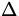N = -Nt, (10)

whereis called the decay constant. Note thathas units of inverse time, or s - 1. The decay rate, R , is defined as the number of decays per unit time:

 R =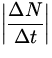=N. (11)

For finite times it is found that the number of nuclei present after a time t is given by

 N = N0e -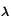t (12)

where N0 is the number of nuclei present at t = 0 and e = 2.71828... is the base of the natural'' logarithms (compared to the base 10 of the common'' logarithms). Thus, from Eq. (29.12), in a time t =a fraction e - 1 = 0.36787944... of a substance remains.

It is sometimes convenient to introduce the half-life, T1/2 , of a substance, defined as the time over which exactly one half of a substance remains. From Eq. (29.12) one finds

 e -T1/2 =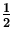T1/2 =(13)

where ln 2 = 0.693... is the natural logarithm of 2 (note ln e = 1 , which is analogous to log 10 = 1 for the common logarithm). In terms of the half-life Eq. (29.12) can be written as=t/T1/2. (14)

Thus, after a period of one half-life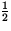of a substance remains, after another half lifex=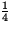of a substance remains, and so on. Half-lives of substances range from tiny fractions of a second to millions of years.Next: Nuclear Reactions Up: Nuclear Physics Previous: Radioactivity